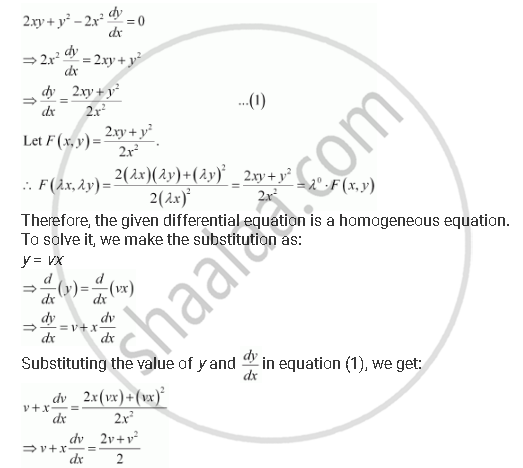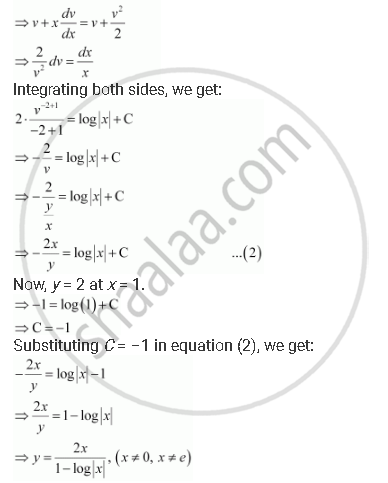Share

# For the Differential Equations Find the Particular Solution Satisfying the Given Condition: 2xy + Y^2 - 2x^2 Dy/Dx = 0; Y = 2 When X = 1 - CBSE (Science) Class 12 - Mathematics

ConceptMethods of Solving First Order, First Degree Differential Equations Homogeneous Differential Equations

#### Question

For the differential equations find the particular solution satisfying the given condition:

2xy + y^2 - 2x^2  dy/dx = 0; y = 2  " when x " = 1

#### SolutionThis is the required solution of the given differential equation.

Is there an error in this question or solution?

#### APPEARS IN

NCERT Solution for Mathematics Textbook for Class 12 (2018 to Current)
Chapter 9: Differential Equations
Q: 15 | Page no. 406
Solution For the Differential Equations Find the Particular Solution Satisfying the Given Condition: 2xy + Y^2 - 2x^2 Dy/Dx = 0; Y = 2 When X = 1 Concept: Methods of Solving First Order, First Degree Differential Equations - Homogeneous Differential Equations.
S